# NCERT Solutions: Decimals- 1 Notes | Study Mathematics (Maths) Class 6 - Class 6

## Class 6: NCERT Solutions: Decimals- 1 Notes | Study Mathematics (Maths) Class 6 - Class 6

The document NCERT Solutions: Decimals- 1 Notes | Study Mathematics (Maths) Class 6 - Class 6 is a part of the Class 6 Course Mathematics (Maths) Class 6.
All you need of Class 6 at this link: Class 6

Exercise 8.1

Question 1: Write the following numbers in the given table.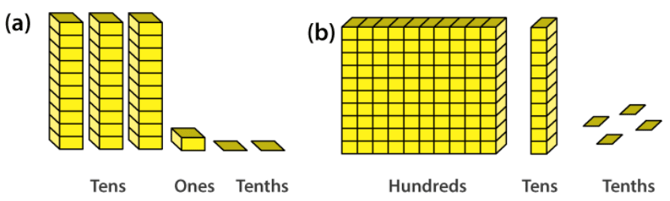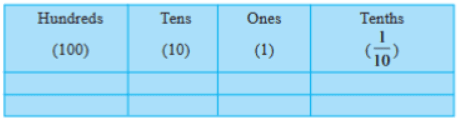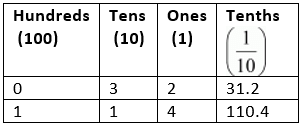Question 2: Write the following decimals in the place value table.
(a) 19.4
(b) 0.3
(c) 10.6
(d) 205.9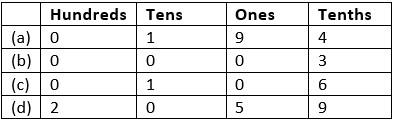Question 3: Write each of the following as decimals:
(a) Seven-tenths
(b) Two tens and nine-tenths
(c) Fourteen point six
(d) One hundred and two ones
(e) Six hundred point eight
(a) seven-tenths = 7/10 = 0.7
(b) 2 tens and 9-tenths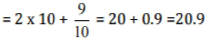(c) Fourteen point six = 14.6
(d) One hundred and 2-ones = 100 + 2 x 1 = 100 + 2 = 102
(e) Six hundred point eight = 600.8

Question 4: Write each of the following as decimals:
(a) 5/10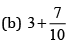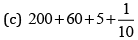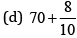(e) 88/10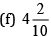(g) 3/2
(h) 2/5
(i) 12/5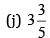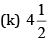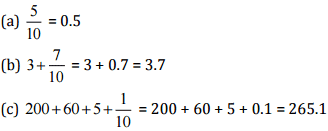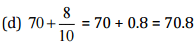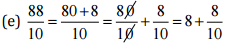= 8 + 0.8 = 8.8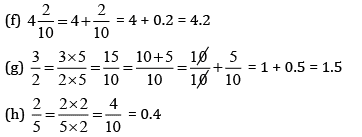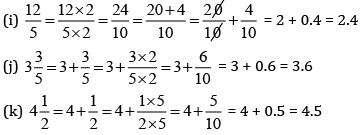Question 5: Write the following decimals as fractions. Reduce the fractions to lowest terms.
(a) 0.6
(b) 2.5
(c) 1.0
(d) 3.8
(e) 13.7
(f) 21.2
(g) 6.4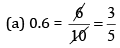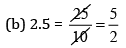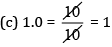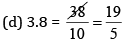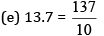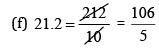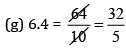Question 6: Express the following as cm using decimals.
(a) 2 mm
(b) 30 mm
(c) 116 mm
(d) 4 cm 2 mm
(e) 162 mm
(f) 83 mm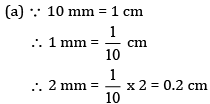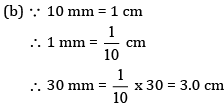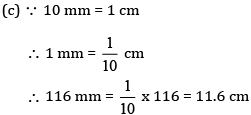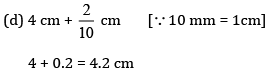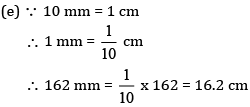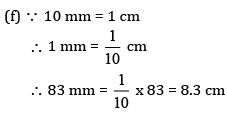Question 7: Between which two whole numbers on the number line are the given lie? Which of these whole numbers is nearer the number?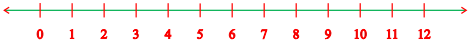(a) 0.8
(b) 5.1
(c) 2.6
(d) 6.4
(e) 9.1
(f) 4.9
(a) From 0 to 1, 0.8 is nearest to 1.
(b) From 5 to 6, 5.1 is nearest to 5.
(c) From 2 to 3, 2.6 is nearest to 3.
(d) From 6 to 7, 6.4 is nearest to 6.
(e) From 9 to 10, 9.1 is nearest to 9.
(f) From 4 to 5, 4.9 is nearest to 5.

Question 8: Show the following numbers on the number line:
(a) 0.2
(b) 1.9
(c) 1.1
(d) 2.5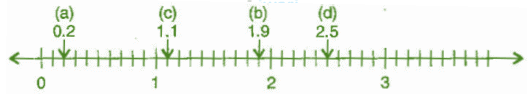Question 9: Write the decimal number represented by the points A, B, C, D on the given number line.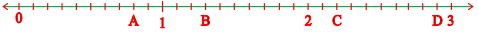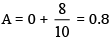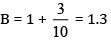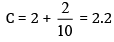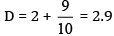Question 10:
(a) The length of Ramesh’s notebook is 9 cm and 5 mm. What will be its length in cm?
(b) The length of a young gram plant is 65 mm. Express its length in cm.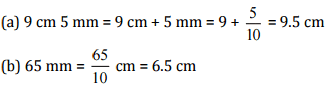Exercise 8.2

Question 1: Complete the table with the help of these boxes and use decimals to write the number.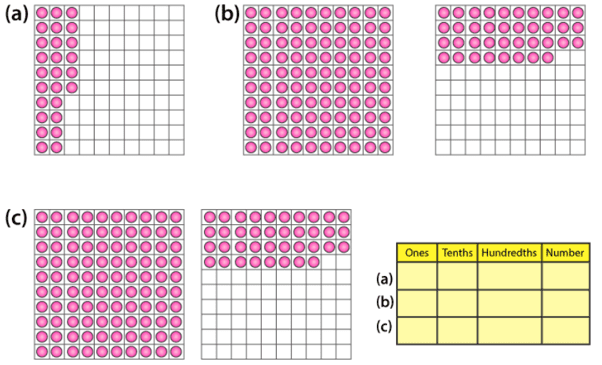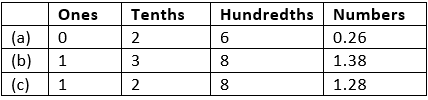Question 2: Write the numbers given in the following place value table in decimal form.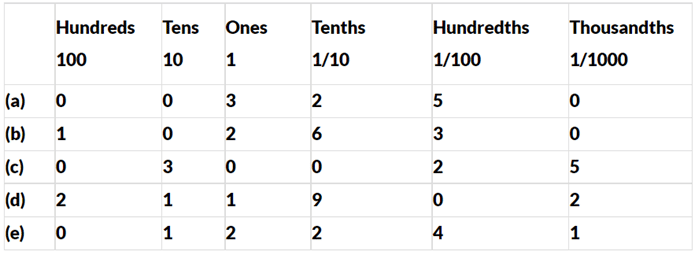(a)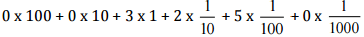= 0 + 0 + 3 + 0.2 + 0.05 + 0 = 3.25
(b) 1 x 100 + 0 x 10 + 2 x 1 + 6 x (1/10) + 3 x 1/100 + 0 x (1/1000)
= 1 + 0 + 2 + 0.6 + 0.03 + 0 = 102.63
(c) 0 x 100 + 3 x 10 + 0 x 1 + 0 x (1/10) + 2 x 1/100 + 5 x (1/1000)
= 0 + 30 + 0 + 0 + 0.02 + 0.005 = 30.025
(d) 2 x 100 + 1 x 10 + 1 x 1 + 9 x 1/10 + 0 x 1/100 + 2 x (1/1000)
= 200 + 10 + 1 + 0.9 + 0 + 0.002 = 211.902
(e) 0 x 100 + 1 x 10 + 2 x 1 + 2 x 1/10 + 4 x 1/100 + 1 x (1/1000)
= 0 + 10 + 2 + 0.2 + 0.04 + 0.001 = 12.241

Question 3: Write the following decimals in the place value table:
(a) 0.29
(b) 2.08
(c) 19.60
(d) 148.32
(e) 200.812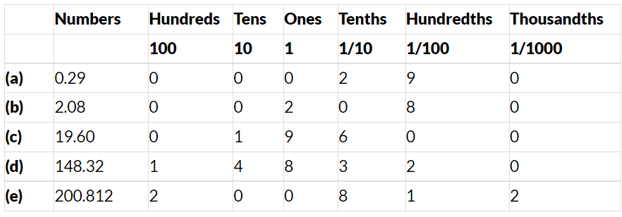Question 4: Write each of the following as decimals:
(a)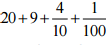(b) 137 + (5/100)
(c)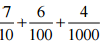(d)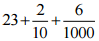(e)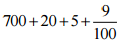(a) 20 + 9 + 0.4 + 0.01 = 29.41
(b) 137 + 0.05 = 137.05
(c) 0.7 + 0.06 + 0.004 = 0.764
(d) 23 + 0.2 + 0.006 = 23.206
(e) 700 + 20 + 5 + 0.09 = 725.09

Question 5: Write each of the following decimals in words:
(a) 0.03
(b) 1.20
(c) 108.56
(d) 10.07
(e) 0.032
(f) 5.008
(a) Zero point zero three
(b) One point two zero
(c) One hundred and eight point five six
(d) Ten point zero seven
(e) Zero point zero three two
(f) Five point zero zero eight

Question 6: Between which two numbers in tenths place on the number line does each of the given number lie?
(a) 0.06
(b) 0.45
(c) 0.19
(d) 0.66
(e) 0.92
(f) 0.57

Answer 6: (a) 0.60 lies between 0 and 0.1 in tenths place
(b) 0.45 lies between 0.4 and 0.5 in tenths place
(c) 0.19 lies between 0.1 and 0.2 in tenths place
(d) 0.66 lies between 0.6 and 0.7 in tenths place
(e) 0.92 lies between 0.9 and 1.0 in tenths place
(f) 0.57 lies between 0.5 and 0.6 in tenths place

Question 7: Write as fractions in lowest terms:
(a) 0.60
(b) 0.05
(c) 0.75
(d) 0.18
(e) 0.25
(f) 0.125
(g) 0.066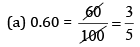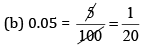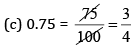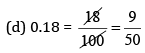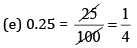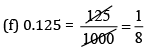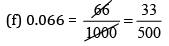The document NCERT Solutions: Decimals- 1 Notes | Study Mathematics (Maths) Class 6 - Class 6 is a part of the Class 6 Course Mathematics (Maths) Class 6.
All you need of Class 6 at this link: Class 6Use Code STAYHOME200 and get INR 200 additional OFF

## Mathematics (Maths) Class 6

239 videos|245 docs|43 tests

Track your progress, build streaks, highlight & save important lessons and more!

,

,

,

,

,

,

,

,

,

,

,

,

,

,

,

,

,

,

,

,

,

;